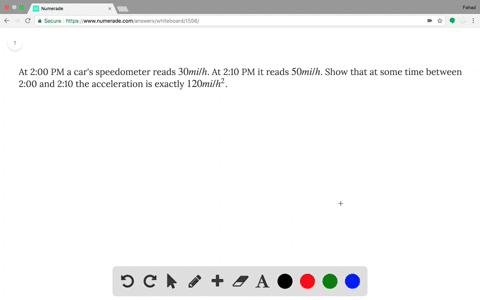Enroll in one of our FREE online STEM summer camps. Space is limited so join now!View Summer Courses### At 2:00 PM a car's speedometer reads $30 mi/h$.…

03:33University of North Texas

Need more help? Fill out this quick form to get professional live tutoring.

Get live tutoring
Problem 35

Prove the identity $\arcsin \frac{x - 1}{x + 1} = 2\arctan \sqrt{x} - \frac{\pi}{2}$.

Let $f(x)=\arcsin \left(\frac{x-1}{x+1}\right)-2 \arctan \sqrt{x}+\frac{\pi}{2} .$ Note that the domain of $f$ is $[0, \infty) .$ Thus
$f^{\prime}(x)=\frac{1}{\sqrt{1-\left(\frac{x-1}{x+1}\right)^{2}}} \frac{(x+1)-(x-1)}{(x+1)^{2}}-\frac{2}{1+x} \cdot \frac{1}{2 \sqrt{x}}=\frac{1}{\sqrt{x}(x+1)}-\frac{1}{\sqrt{x}(x+1)}=0$
Then $f(x)=C$ on $(0, \infty)$ by Theorem $5 .$ By continuity of $f, f(x)=C$ on $[0, \infty) .$ To find $C,$ we let $x=0 \Rightarrow$
$\begin{array}{l} \arcsin (-1)-2 \arctan (0)+\frac{\pi}{2}=C \Rightarrow-\frac{\pi}{2}-0+\frac{\pi}{2}=0=C \cdot \text { Thus, } f(x)=0 \Rightarrow \\ \arcsin \left(\frac{x-1}{x+1}\right)=2 \arctan \sqrt{x}-\frac{\pi}{2} \end{array}$

## Discussion

You must be signed in to discuss.

## Video Transcript

So what we want to do is prove that the identity arc sine of X minus one over explicit one secret to two times arc tangent of the square root of X minus pi half So something that will help us with this. I went ahead and define two functions f of X as park sign of X minus one over X plus one and g of X as two times our attention of the square root of X. And the reason why I want to do this is because both of their derivatives ends up being the same Attn least when we'd look, um, on the interval. So I should say, on zero to infinity, these have the same derivatives, and that's gonna kind of coming toe play to help us later. All right, Uh, so what we're going to want to do now is let's define 1/3 function, and I'm gonna call this one a trucks, and what we're going to do is look this be defined as f of X minus G of X. Now, the reason why we want to do this is if we were to go ahead and take the derivative of H of X. This here? Well, give us the derivative of that. So we get the derivative of X minus two derivative defects. And the more important thing is, since both of these have the same derivative bowl, why subtract thes? I just end up with zero. Now, the thing that is more so important about this is, since this is true on it interval for every value of X on zero to infinity, that h of X is constant. So with this implies is that h of X is constant on zero to infinity. I remember this is the case. Ah, by one of the serums in our, um in this chapter now, there might be a nice value on zero infinity that we can plug into H to Europe with this constant should be end. It just so happens to be that each of one will be a nice value for us to pluck it. So let's go ahead and so is gonna be a chav. One is equal to half of one minus G F one and then we go had implode one into F, which is going to be arc sign of one minus one over one plus one minus two times are Tanja of the square root of one. So we end up with arc sine of well, one minus 10 So we just have Mark sign of zero minus two arc tangent of one. So arc sine of zero is going to be zero and then arc Tangent of one is pie forth. So now we can go ahead and simplify this down to negative pie half. So what we have is that h of X is equal to negative pie half. So if we go ahead and rewrite this as f of X minus g of X, so we have f of X minus G of X is able to negative pie half will be add g of X over. Now we get f of X is equal to G of X minus pi, huh? And then, if we plug in what f of X is so f of x waas arc sine of X minus one over X plus one and G of X was to arc tangent of the square root of X, And then we still have that minus pi half. And so this here is what we wanted to show. So since we finished our proof, we can parallel proof box and mail spotted face. So the idea when we're trying to do these normally is we look for two functions that have the same derivative. We can track them get zero, and then we apply that fear. Um, that says since the derivative is always zero, that means h of X has to be a constant on that interval.